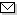Language:   Search:   ContactSearch and Browse the MSC 2000

Search:
Please note that in the second half of 2009, MSC2010 is deployed in the production of ZMATH and will become official standard in January 2010.

Enter a query and click »Search«...

45-XX Integral equations → ZMATH
45-00 General reference works (handbooks, dictionaries, bibliographies, etc.) → ZMATH
45-01 Instructional exposition (textbooks, tutorial papers, etc.) → ZMATH
45-02 Research exposition (monographs, survey articles) → ZMATH
45-03 Historical (must also be assigned at least one classification number from Section 01) → ZMATH
45-04 Explicit machine computation and programs (not the theory of computation or programming) → ZMATH
45-06 Proceedings, conferences, collections, etc. → ZMATH
45A05 Linear integral equations → ZMATH
45B05 Fredholm integral equations → ZMATH
45C05 Eigenvalue problems [See also 34Lxx, 35Pxx, 45P05, 47A75] → ZMATH
45D05 Volterra integral equations [See also 34A12] → ZMATH
45Exx Singular integral equations [See also 30Exx, 44-XX, 30E20, 30E25, 44A15, 44A35] → ZMATH
45Fxx Systems of linear integral equations → ZMATH
45Gxx Nonlinear integral equations [See also 47H30, 47Jxx] → ZMATH
45H05 Miscellaneous special kernels [See also 44A15] → ZMATH
45J05 Integro-ordinary differential equations [See also 34K05, 34K30, 47G20] → ZMATH
45K05 Integro-partial differential equations [See also 34K30, 35R10, 47G20] → ZMATH
45L05 Theoretical approximation of solutions {For numerical analysis, see 65Rxx} → ZMATH
45Mxx Qualitative behavior → ZMATH
45N05 Abstract integral equations, integral equations in abstract spaces → ZMATH
45P05 Integral operators [See also 47B38, 47G10] → ZMATH
45Q05 Inverse problems → ZMATH
45R05 Random integral equations [See also 60H20] → ZMATH

NewsAbel prize 2010I. M. Gelfand 1913-2009MSC2010

Copyright © 2019 Zentralblatt MATH | European Mathematical Society | FIZ Karlsruhe | Heidelberg Academy of Sciences